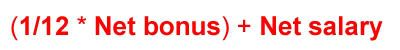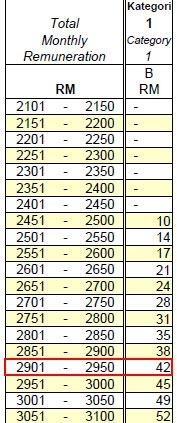# How To Calculate PCB for Bonus?

Most of the people will have doubt on how to calculate the PCB for bonus. It’s always best for us to know how the PCB is calculated so that we can plan ahead of our future money. I found that quite a great amount of my year end bonus has deducted. After I figured out, I only know that there’s a formula to calculate PCB for bonus.The Potongan Cukai Bulanan (PCB) calculation for bonus is not that straight forward. Here’s how PCB for bonus is calculated:

Let say your monthly salary is RM3,000, you are contributing 11% from your salary to your EPF savings, and you received 1 month bonus in January 2011.

1 month bonus = RM3,000
EPF deduction = RM330
Net Salary = RM2,670
Maximum total EPF deduction allowed for PCB calculation is only RM500. So, maximum available EPF deduction for PCB calculation = RM500 – RM330 = RM170

Bonus to calculate PCB
= Gross bonus – EPF deduction left
= RM3,000 – RM170
= RM2,830

The formula to calculate the PCB for bonus:Thus, amount
= (1/12 * RM2,830) + RM2,670
= RM2,905.83Based on Jadual PCB 2010, the monthly tax deduction for RM2,905.83 is RM42.

Total PCB for bonus = RM42 * 12 = RM216

Therefore, for the month with bonus:
Bonus = RM3,000
EPF contribution from employee, 11% = RM330
EPF contribution from employer, 12% = RM360
SOCSO contribution from employee = RM14.75
SOCSO contribution from employer = RM51.65
PCB deduction = RM216

Thus, the amount that you will get = RM3,000 – (RM330 + RM14.75 + RM216)= RM2439.25

With the formula provided, now you can try to calculate your received bonus and let me know whether the calculation and formula is accurate.

## 2 thoughts on “How To Calculate PCB for Bonus?”

1.guest says:

2.Rikishi says: